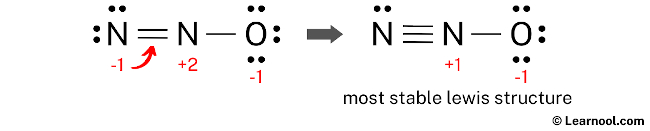# N2O Lewis structure

N2O (nitrous oxide) has two nitrogen atoms and one oxygen atom.

In the N2O Lewis structure, there is a triple bond between two nitrogen atoms, and a single bond between nitrogen and oxygen atom. The left nitrogen atom with a triple bond has one lone pair, and the oxygen atom with a single bond has three lone pairs.

Also, there is a positive (+1) charge on the center nitrogen atom, and a negative (-1) charge on the oxygen atom.

Contents

## Steps

Here’s how you can easily draw the N2O Lewis structure step by step:

#1 Draw a rough skeleton structure
#2 Mention lone pairs on the atoms
#3 If needed, mention formal charges on the atoms
#4 Minimize formal charges by converting lone pairs of the atoms, and try to get a stable Lewis structure
#5 Repeat step 4 again if needed, until all charges are minimized

Now, let’s take a closer look at each step mentioned above.

### #1 Draw a rough skeleton structure

• First, determine the total number of valence electrons

In the periodic table, nitrogen lies in group 15, and oxygen lies in group 16.

Hence, nitrogen has five valence electrons and oxygen has six valence electrons.

Since N2O has two nitrogen atoms and one oxygen atom, so…

Valence electrons of two nitrogen atoms = 5 × 2 = 10
Valence electrons of one oxygen atom = 6 × 1 = 6

And the total valence electrons = 10 + 6 = 16

• Second, find the total electron pairs

We have a total of 16 valence electrons. And when we divide this value by two, we get the value of total electron pairs.

Total electron pairs = total valence electrons ÷ 2

So the total electron pairs = 16 ÷ 2 = 8

• Third, determine the central atom

We have to place the least electronegative atom at the center.

Since nitrogen is less electronegative than oxygen, assume that the central atom is nitrogen.

Here, there are two nitrogen atoms, so we can assume any one as the central atom.

Let’s assume that the central atom is center nitrogen.

• And finally, draw the rough sketch

### #2 Mention lone pairs on the atoms

Here, we have a total of 8 electron pairs. And two bonds are already marked. So we have to only mark the remaining six electron pairs as lone pairs on the sketch.

Also remember that both (nitrogen and oxygen) are the period 2 elements, so they can not keep more than 8 electrons in their last shell.

Always start to mark the lone pairs from outside atoms. Here, the outside atoms are left nitrogen and oxygen.

So for left nitrogen and oxygen, there are three lone pairs, and for center nitrogen, there is zero lone pair because all six electron pairs are over.

Mark the lone pairs on the sketch as follows:

### #3 If needed, mention formal charges on the atoms

Use the following formula to calculate the formal charges on atoms:

Formal charge = valence electrons – nonbonding electrons – ½ bonding electrons

For left nitrogen atom, formal charge = 5 – 6 – ½ (2) = -2

For center nitrogen atom, formal charge = 5 – 0 – ½ (4) = +3

For oxygen atom, formal charge = 6 – 6 – ½ (2) = -1

Here, both nitrogen and oxygen atoms have charges, so mark them on the sketch as follows:

The above structure is not a stable Lewis structure because both nitrogen and oxygen atoms have charges. Therefore, reduce the charges (as below) by converting lone pairs to bonds.

### #4 Minimize formal charges by converting lone pairs of the atoms

Here we have to convert a lone pair of the left nitrogen atom (instead of the oxygen atom). Because nitrogen is less electronegative than oxygen. And so, it can give more valence electrons to share them.

Or remember this way, if we convert a lone pair of the left nitrogen atom (instead of the oxygen atom), then…

1) we get the formal charges on atoms closer to zero.
2) the negative (-1) charge comes on the most electronegative element. In this case, the most electronegative element is oxygen.

Therefore, convert a lone pair of the left nitrogen atom to make a new N — N bond with the center nitrogen atom as follows:

### #5 Since there are charges on atoms, repeat step 4 again

Since there are charges on nitrogen and oxygen atoms, again convert a lone pair of the left nitrogen atom to make a new N — N bond with the center nitrogen atom as follows:Lone pair of left nitrogen is converted again, and got the most stable Lewis structure of N2O

In the above structure, you can see that the central atom (center nitrogen) forms an octet. And the outside atoms (left nitrogen and oxygen) also form an octet. Hence, the octet rule is satisfied.

Now there are still charges on the atoms. But we can not convert a lone pair to a bond because nitrogen can not keep more than 8 electrons in its last shell.

The formal charges on atoms are closer to zero. Also, the above structure is more stable than the previous structures. Therefore, this structure is the most stable Lewis structure of N2O.